# 3.6 Mechanical Stress

If several materials are combined within a microstructure, mechanical forces occur if the volumes of the different materials change. Several effects may enforce volume expansion, for instance chemical reactions, phase interchanges and recrystallization, mass migration, and thermal expansion and contraction. In this thesis, the mechanical stress due to thermal expansion is considered. The mechanical stress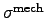can be separated into a static and a thermal stress component  as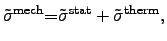(3.23)

where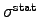represents the static stress component, which is impressed and mostly fixed by the fabrication processes, for instance material deposition, annealing, and packaging. The thermal component of the stress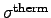is a transient quantity influenced by the ambient temperature and other state variables of the system. The corresponding hydrostatic pressure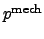is then defined as the trace of the mechanical stress tensor,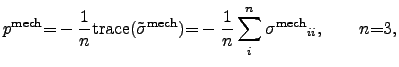for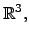(3.24)

which represents an averaged value and can be used for significant comparisons, as a figure of merit for optimization purposes, and to visualize the mechanical stress in a microelectronic device structure.

The local force density which acts on the material can be described by the mechanical stress tensor, which can be derived from the mechanical strain tensorusing the LAMÉ3.4 formalism to model (2.109) as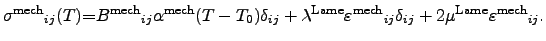(3.25)

The quantities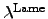and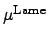are the LAMÉ constants, which can be expressed by YOUNG's modulus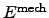and POISSON's ratio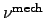[96,97] as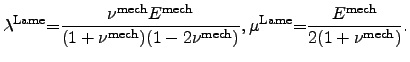(3.26)

For orthotropic materials the number of independent components for the stiffness and stress tensor is reduced due to energetic considerations and symmetry in the crystals [202,96,97]. Hence, the number of independent components of the stiffness tensor (forth rank) is reduced to nine and for the stress tensor (second rank) to six. Hence, the mechanical problem can be expressed in terms of vectors and matrices  according to the VOIGT notation.

Stefan Holzer 2007-11-19